Courses

# Unit 4: Average Due Date CA CPT Notes | EduRev

## CA CPT : Unit 4: Average Due Date CA CPT Notes | EduRev

The document Unit 4: Average Due Date CA CPT Notes | EduRev is a part of the CA CPT Course Principles and Practice of Accounting.
All you need of CA CPT at this link: CA CPT

4.1 Introduction
In business enterprises, a large number of receipts and payments by and from a single party may occur at different points of time. To simplify the calculation of interest involved for such transactions, the idea of average due date has been developed. Where a person owing several amounts due on different dates, desires to pay the total amount payable by him/her on a particular date, so that neither the debtor nor the creditor stands to lose or gain anything by way of interest, that date is known as average due date. Average Due Date is weighted average of due dates of various transactions where amount of each transaction is used as weight. The unique feature f this approach is that the party making payment neither suffers any loss nor gains anything by this arrangement of making a single payment. Average due date is generally used in following circumstances:

i) For calculating interest on drawings of partners;
ii) For settling accounts between principle and agent;
iii) For settling contra accounts e.g. where parties sell goods to each other;
iv) For making lump sum payment against various bills drawn on different dates with different due dates;
In this unit, we shall elaborate the underlying principle of determining average due date covering the cases where the amount is lent in various instalments but repayment is made in a single instalment as well as where the amount is lent in one instalment but repayment is made by various instalments. The technique of average due date is also useful for calculating interest on drawings made by the proprietors or partners of a business firm at several points of time.
Average due date: It is the mean or equated date on which a single total payment may be made in lieu of different payments on different dates without any loss to either party. Where payment is not made on the average due date, the party receiving the amount charges interest for as many days as the payment is delayed from the average due date.
The formula for calculating average due date is as follows: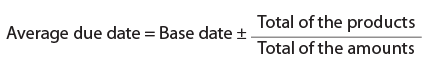Points to be noted:
1. Selection of base date/ zero date: Such a date may be the due date of the first transaction or the due date of the last transaction or any other due date between the first and the last but preferably earlier due date may be taken.
2. While ascertaining the number of intervening days (plus or minus) between the base date and the due date of each transaction ignore the first date and include the last day.
3. If due date is in fraction, round it off.
4. If amount is paid before due date, rebate is given.
5. If amount is paid after due date, then interest is charged.
6. Whenever there is a sale of goods by two persons to each other on different dates, the formula for calculating average due date becomes: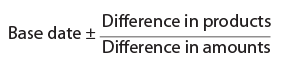4.2 Concept of Due Date (Date of Maturity)
The due date of a bill of exchange/invoice is the date when the amount of a bill/invoice is payable by the drawee/ creditor to drawer/ debtor.

4.2.1 Calculation of Due Date after Taking into Consideration Days of Grace
A Bill of exchange or promissory note matures on the date on which it falls due. And every promissory note or bill of exchange (other than those payable on demand or at sight or on presentment) falls due on the third day after on which it is expressed to be payable.
Examples
(i) A bill dated 30th September is made payable three months after date. It falls due on 2nd January.
(ii) Due Date=30 Dec
(iii) Maturity date= 30 Dec +3 =2 Jan
(iv) A note dated 1st January is payable one month after sight. It falls due on 4th February.

4.2.2 Calculating Due Date of Bill or Note Payable Few Months after Date or Sight
When the bill is made payable at a stated number of months after date or after sight or after certain events, then the period stated shall be held to terminate on the date of the month which corresponds with the day on which the instrument is dated. If the month in which the period would terminate has no corresponding day, the period shall be held to terminate on the last day of such month.
Example: A Bill due on 29th January, 2015 is made payable at one month after date. The due date of instrument is 3rd day after 28th February, i.e., 3rd March (in 2015, February is of 28 days only).

4.2.3 Calculation of Due Date when the Maturity Day is a Holiday
When the day on which a promissory note or bill of exchange is at maturity (after including days of grace) is a public holiday, the instrument shall be deemed to be due on the preceding business day. The expression "public holiday" includes Sundays and other days declared by the Central Government by notification in the official gazette, to be a public holiday. And now if the preceding day is also a public holiday, it will fall on the day preceding the previous day. But if the holiday happens to be emergency or unforeseen holiday then the date shall be the next following day.(Ref: Negotiable Instruments Act 1881).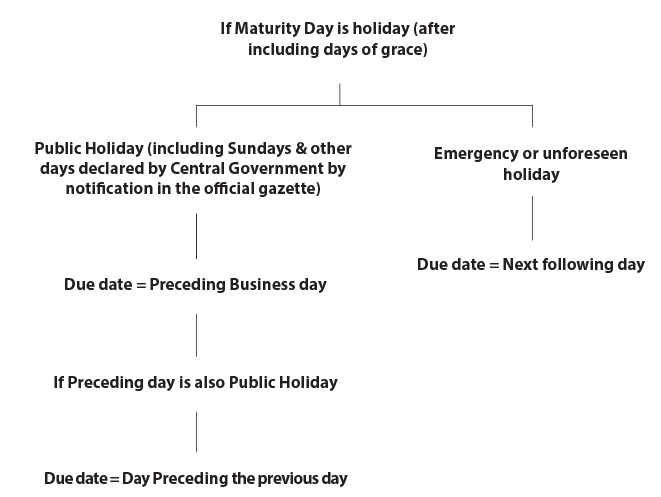4.3 Types of Problems
♦ Case 1: Learn calculation of average due date where one Party is involved
♦ Case 2: Learn calculation of average due date where inter transactions between 2 Parties are involved
♦ Case 3: Learn calculation of average due date where amount is repaid in Instalments
♦ Case 4: Learn Calculation of average due date for determining interest on drawings.

Case 1: Learn calculation of average due date Where one Party is involved Calculation of average due date
Under this type of problem, average due date is calculated as follows :
a. Take the earliest due date as starting day or base date or "O" day for convenience. Any date whatsoever, may also be taken as "O" day.
b. Consider the number of days from base date up to each due date. Calculations may also be made in month.
c. Multiply the number of days by the corresponding amounts.
d. Add up the amount and products.
e. Divide the "Product total" by "Amount total" and get result approximately upto a whole number.
f. This number is added in the base date to find the average due date.
Thus the formula for the average due date can be under.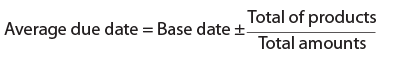Note: For calculation of no. of days, no. of days in each respective month involved are to be considered individually.

ILLUSTRATION 1
The followings are the amounts due on different dates in between the same parties: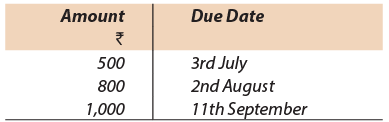Suggest a date on which all the bills may be paid out without any loss of interest to either party.
Solution:
Considering 3rd July as the starting day the following table is prepared: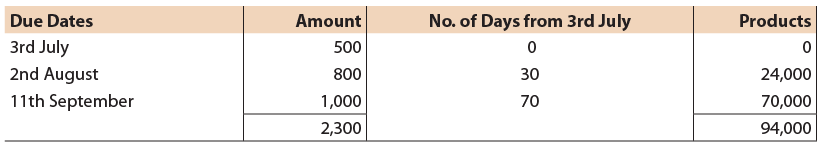Loss of Interest: 13th August to 11th September
Assuming 5% is interest rate, the debtor loses interest due to early payment of ₹ 1,000 for 29 days (from 13th August to 11th September) i.e., ₹ 4. 1000 x 29/365 x 5/100
Gain of Interest: 3rd July to 13th August and 2 August to 13th August
He however, gains interest, due to late payment on ₹ 500 for 41 days from 3rd July to 13th August and on ₹ 800 for 11 days i.e. ₹ 2.80 + ₹ 1.20, i.e., ₹ 4.
Thus, the debtor neither loses nor gains by payment of all the amounts on 13th August.
It should be noted that in calculating the number of days only one of the dates, either the starting date or the due date is to be counted. In the same manner, bill due to one party may be cancelled as against bills of same amount due from the same party after adjustment of interest for the period elapsing between the two average due dates.
Instead of payment of several bills on the same date as above, other bill starting from the average due date for agreed period together with interest for the period may be accepted.

ILLUSTRATION 2
The following amounts are due to X by Y. Y wants to pay off (a) on 18th March or (b) on 14th July. Interest rate of 8% p.a. is taken into consideration.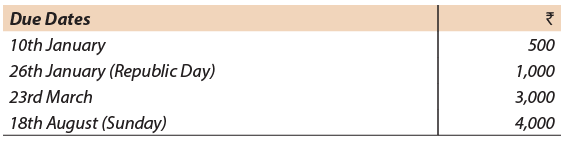Determine the amount to be paid in (a) and in (b).
Solution: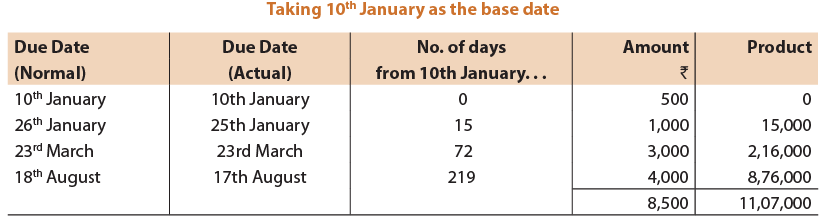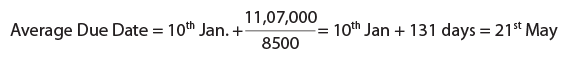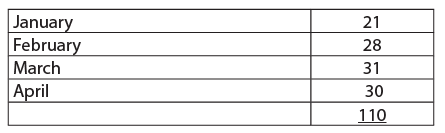(a) If the payment is made on 18th March rebate will be allowed for unexpired time from 18th March to 21th May i.e., 13 + 30 + 21 i.e. for 64 days. He has to pay the discounted value of the total amount.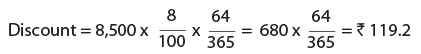(b) If the payment is deferred to 14th July, interest is to be paid from 21th May to 14th July i.e., for 10 + 30 + 14 = 54 days.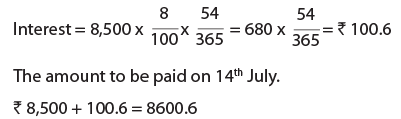ILLUSTRATION 3
Calculate Average Due date from the following information: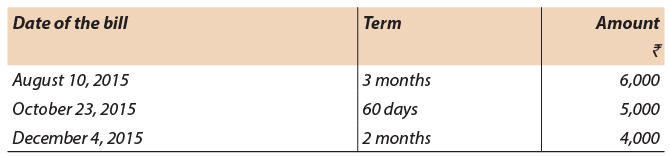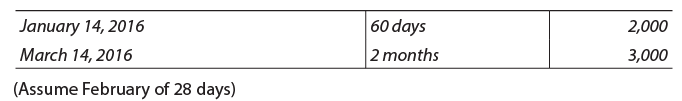Solution:
Calculation of Average Due Date Taking 10th August 2015 as the base date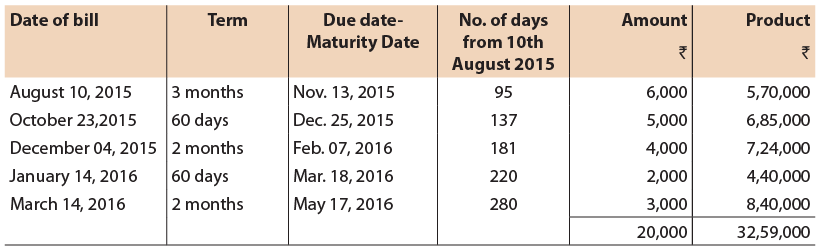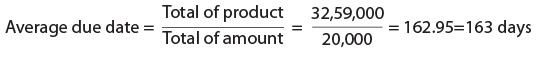= 163 days after August 10, 2015 i.e. January 20, 2016.
Days of Grace added as it is case of Bills and it is Negotiable Instrument.

ILLUSTRATION 4
A trader having accepted the following several bills falling due on dierent dates, now desires to have these bills cancelled and to accept a new bill for the whole amount payable on the average due date :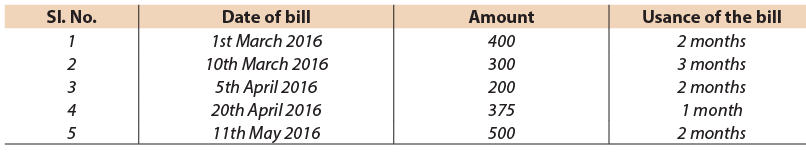You are required to find the said average due date.
Solution:
Calculation of the average due date
Taking 4th May as the base date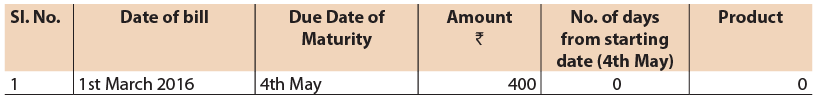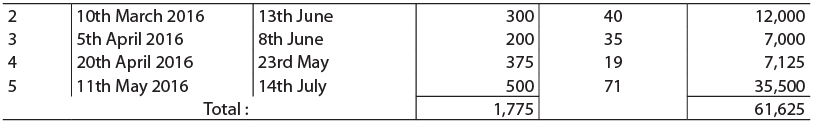Average Due Date is 61,625/1,775=34.71 i.e., 35 days after the assumed due date, 4th May, 2016. The new bill should be for ' 1,775 payable on June 8th, 2016.
Case 2: Learn calculation of average due date Where inter transactions between 2 Parties are involved
When more than one party is involved where one party purchase and also sells to other party like JK Tyres and Maruti where Maruti sells car to JK Tyres for their employees and purchases Tyres from them. In such a case instead of paying gross amount they may go for new amount i.e. Purchase amount and sales amount will be set off and thus here we take difference of amount and produce as Net Amount. In such cases, earliest date of both parties is taken as the base date.

ILLUSTRATION 5
Two traders X and Y buy goods from one another, each allowing the other one month's credit. At the end of 3 months the accounts rendered are as follows: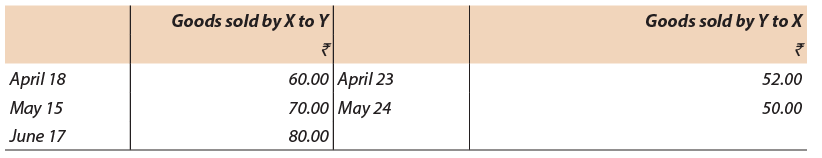Calculate the date upon which the balance should be paid so that no interest is due either to X or Y.
Solution:
Taking May 18th as the zero or base date ( April 18 +One month Credit=18 May)
For Y’s payments: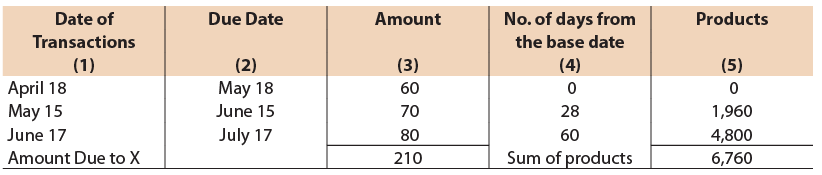For X’s payments
The students should note that the same base date should be taken. Therefore, the base date will be May 18th in this case also.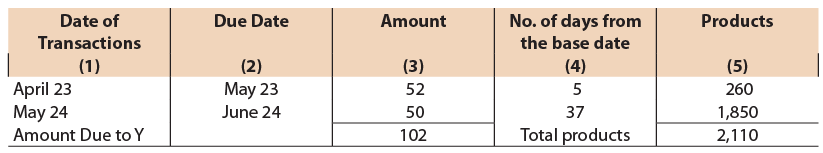Excess of Y’s products over X’s = 6,760 – 2,110
= 4,650
Excess amount due to X ₹ 210 – 102 = ₹ 108.
Number of days from the base date to the date of settlement is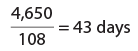Hence the date of settlement of the balance is 43 days after May 18 i.e., on June 30. On June 30, Y has to pay X, ₹ 108 to clear the account.

ILLUSTRATION 6
Manoj had the following bills receivables and bills payable against Sohan. Calculate the average due date, when the payment can be received or made without any loss of interest.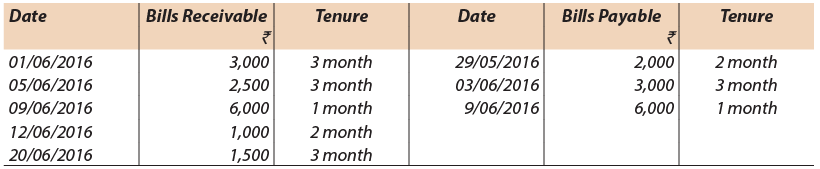15 August, 2016 was a Public holiday. However, 6 September, 2016 was also declared as sudden holiday.
Solution:
Let us take 12.07.2014 as Base date.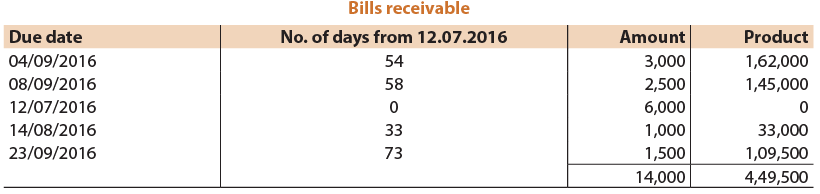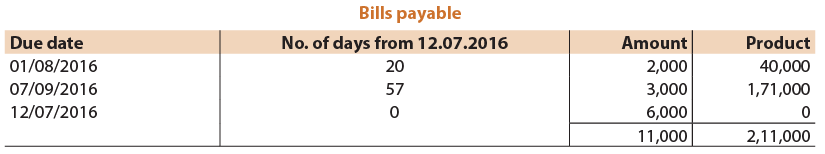Excess of products of bills receivable over bills payable = 4,49,500 -2,11,000= 2,38,500
Excess of bills receivable over bills payable = 14,000 – 11,000 = 3,000
Number of days from the base date to the date of settlement is 2,38,500/3,000 = 79.5 (appox.)
Hence date of settlement of the balance amount is 80 days after 12th July i.e. 30th September.
On 30th September, 2016 Sohan has to pay Manoj ₹ 3,000 to settle the account.

ILLUSTRATION 7
Mr. Green and Mr. Red had the following mutual dealings and desire to settle their account on the average due date:You are asked to ascertain the average due date. ( 28 days in feb.)
Solution:
Calculation of Average Due Date
Taking 6th January, 2016 as base date
For Green’s payments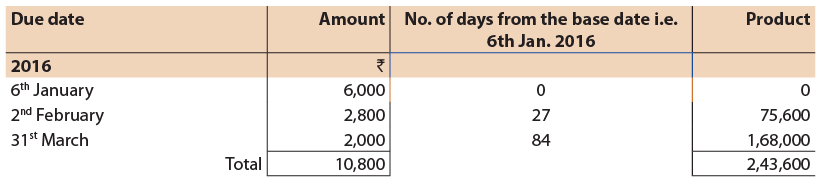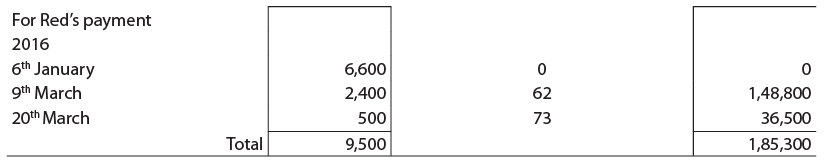Excess of Green’s products over Red’s = ₹ 2,43,600 – ₹ 1,85,300 = ₹ 58,300
= ₹ 10,800 – ₹ 9,500 = ₹ 1,300
Number of days from the base date to the date of settlement is 58,300/1,300=45 days (approx.)
Hence, the date of settlement of the balance amount is 45 days after 6th January i.e. on 20th February.
On 20th  February, 2016, Green has to pay Red ₹ 1,300 to settle the account.
Case 3: Learn calculation of average due date where amount is repaid in Instalments
Calculation of average due date in a case where the amount is lent in one instalment and repayment is done in various instalments (opposite to what we have done in the first case). The problem takes a different shape. The procedure for calculating average due date can be summarized as under:
Step 1: Calculate number of days/monthly/years from the date of lending money to the date of each repayment.
Step 2: Find the total of such days/months/years.
Step 3: Quotient will be the number of days/months/years by which average due date falls away from date of commencement of loan.
As explained earlier, if instalment are same, we can use Simple mean concept i. Divide days by number of items and no need for product.
Thus, the formula for the average due date can be written as under: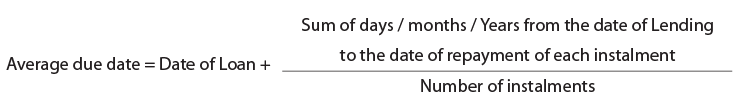ILLUSTRATION 8
₹ 10,000 lent by Dass Bros. to Kumar & Sons on 1st January, 2011 is repayable in 5 equal annual instalments commencing on 1st January, 2012. Find the average due date and calculate interest at 5% per annum, which Dass Bros. will recover from Kumar & Sons.
Solution: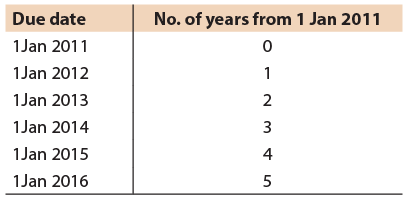Average=5+4+3+2+1/5=3 years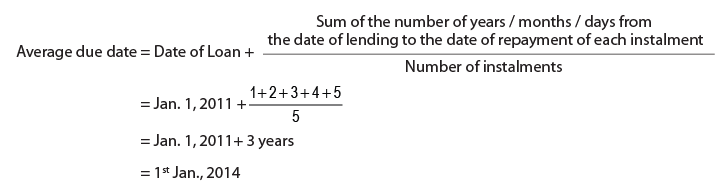Interest at a certain rate on the instalments paid from the date of payment to any fixed date will be the same as on ₹ 10,000 (if lent on 1st Jan., 2014 to that fixed date). There will be no loss to either party. Supposing rate of interest is 5% p.a. and date of settlement is 31st Dec., 2012 then calculation of interest by product method from both parties' point of view will be as follows:
Dass Bros. pays interest as follows: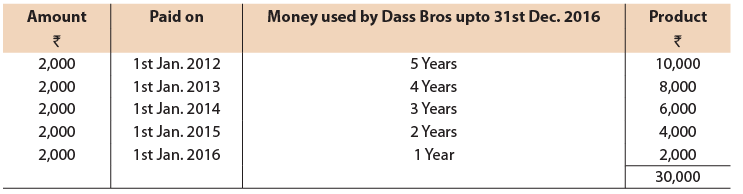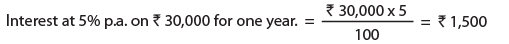Dass Bros. will receive interest (if given on 1st Jan., 2014 on ₹ 10,000 from average due date to 31st Dec.,From the above, it can be concluded that if the borrower pays ₹ 2,000 yearly from 1st Jan., 2012 for 5 years and if the lender gives ₹ 10,000 on 1st Jan., 2014 then both will charge same interest from each other There is no loss to any of the parties. But actually lender gives ₹ 10,000 on 1st Jan., 2011, therefore, he has given loan 3 years in advance and will charge interest on ₹ 10,000 for 3 years.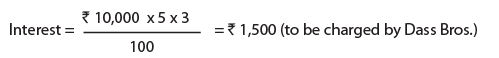Case 4: Learn Calculation of average due date for determining interest on drawings
In the case of drawings also, amount is drawn by the owners of business on various dates but it may settled on one day. It should be noted that, when different amounts are due on different dates, but they are ultimately settled on one day the interest may be calculated by means of Average Due Date. When interest is chargeable on drawings, and drawings are on different dates, interest may be calculated on the basis of Average Due Date of drawings determined on the basis given above. An illustration is given below to help in understanding the same:

ILLUSTRATION 9
A and B, two partners of a firm, have drawn the following amounts from the firm in the year ending 31st March, 2015: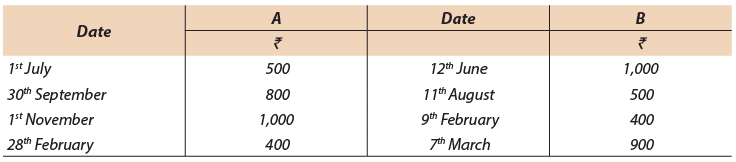Interest at 6% p.a. is charged on all drawings. Calculate interest chargeable by using (i) ordinary system (ii) Average due date system. (assume 1 year = 365 days)
Solution: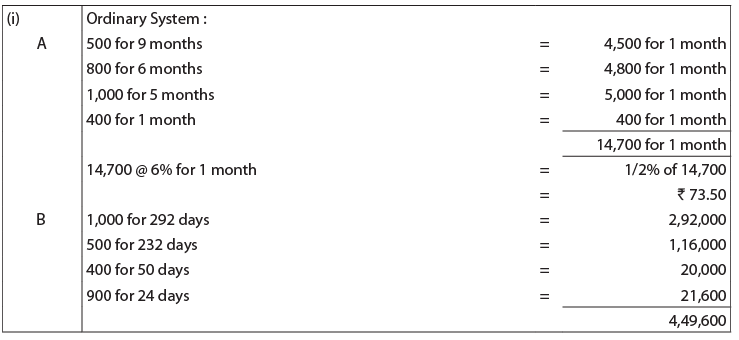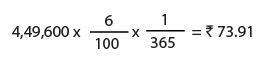(ii) Average Due Date System:
(a) Taking 1st July as the base date (O-day)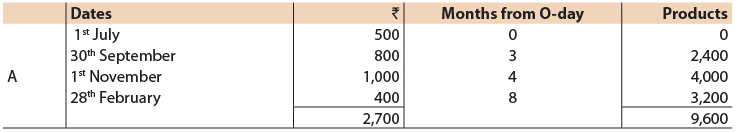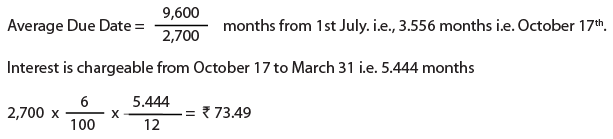Or,
Taking 1st April as the base date (O-day):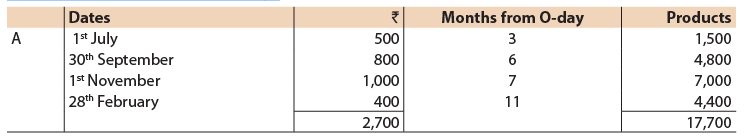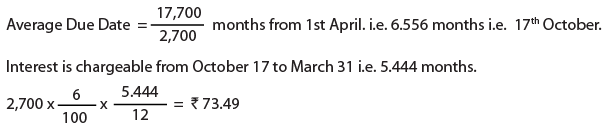(b) Taking 12th June as the base date (Zero-day)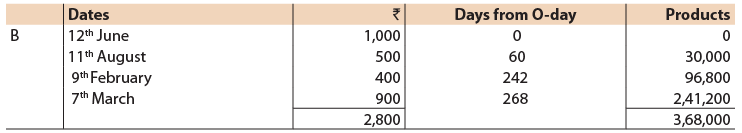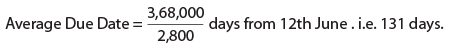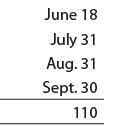131 days -110 days i.e. 21st October
So, interest is chargeable from 21st October to 31st March i.e. for 161 days.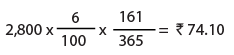The Differences in amounts in the two systems (1) and (2) are due to approximation.

Offer running on EduRev: Apply code STAYHOME200 to get INR 200 off on our premium plan EduRev Infinity!

## Principles and Practice of Accounting

145 docs|79 tests

,

,

,

,

,

,

,

,

,

,

,

,

,

,

,

,

,

,

,

,

,

;